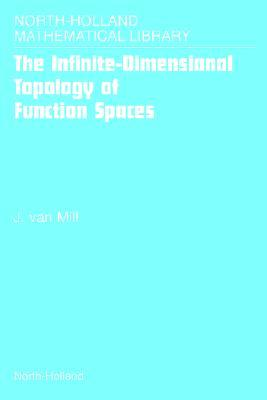Home » The Infinite Dimensional Topology of Function Spacesnorth Holland Mathematical Library Volume 64 by J. van Mill# The Infinite Dimensional Topology of Function Spacesnorth Holland Mathematical Library Volume 64

## J. van Mill

Published May 24th 2002
ISBN : 9780444508492
Paperback
644 pages
Book Rating:Enter the sum

 About the Book In this book we study function spaces of low Borel complexity.Techniques from general topology, infinite-dimensional topology, functional analysis and descriptive set theoryare primarily used for the study of these spaces. The mix ofmethods from several disciplines makes the subjectparticularly interesting. Among other things, a complete and self-contained proof of the Dobrowolski-Marciszewski-Mogilski Theorem that all function spaces of low Borel complexity are topologically homeomorphic, is presented.In order to understand what is going on, a solid background ininfinite-dimensional topology is needed. And for that a fair amount of knowledge of dimension theory as well as ANR theory is needed. The necessary material was partially covered in our previous book Infinite-dimensional topology, prerequisites and introduction. A selection of what was done there can be found here as well, but completely revised and at many places expanded with recent results. A scenic route has been chosen towards theDobrowolski-Marciszewski-Mogilski Theorem, linking theresults needed for its proof to interesting recent research developments in dimension theory and infinite-dimensional topology.The first five chapters of this book are intended as a text forgraduate courses in topology. For a course in dimension theory, Chapters 2 and 3 and part of Chapter 1 should be covered. For a course in infinite-dimensional topology, Chapters 1, 4 and 5. In Chapter 6, which deals with function spaces, recent research results are discussed. It could also be used for a graduate course in topology but its flavor is more that of a research monograph than of a textbook- it is thereforemore suitable as a text for a research seminar. The bookconsequently has the character of both textbook and a research monograph. In Chapters 1 through 5, unless statedotherwise, all spaces under discussion are separable andmetrizable. In Chapter 6 results for more general classes of spaces are presented.In Appendix A for easy reference and some basic facts that are important in the book have been collected. The book is not intended as a basis for a course in topology- its purpose is to collect knowledge about general topology.The exercises in the book serve three purposes: 1) to test the readers understanding of the material 2) to supply proofs of statements that are used in the text, but are not proven there3) to provide additional information not covered by the text.Solutions to selected exercises have been included in Appendix B.These exercises are important or difficult.